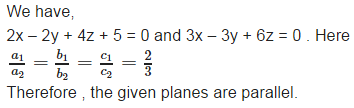# Test: Three Dimensional Geometry (CBSE Level) - 1

## 25 Questions MCQ Test Mathematics (Maths) Class 12 | Test: Three Dimensional Geometry (CBSE Level) - 1

Description
This mock test of Test: Three Dimensional Geometry (CBSE Level) - 1 for JEE helps you for every JEE entrance exam. This contains 25 Multiple Choice Questions for JEE Test: Three Dimensional Geometry (CBSE Level) - 1 (mcq) to study with solutions a complete question bank. The solved questions answers in this Test: Three Dimensional Geometry (CBSE Level) - 1 quiz give you a good mix of easy questions and tough questions. JEE students definitely take this Test: Three Dimensional Geometry (CBSE Level) - 1 exercise for a better result in the exam. You can find other Test: Three Dimensional Geometry (CBSE Level) - 1 extra questions, long questions & short questions for JEE on EduRev as well by searching above.
QUESTION: 1

### Direction cosines of a line are

Solution:

Direction cosines of a line are the cosines of the angles made by the line with the positive direction of the coordinate axis.i.e. x- axis , y-axis and z – axis respectively.

QUESTION: 2

### Shortest distance between two skew lines is

Solution:

Shortest distance between two skew lines is The line segment perpendicular to both the lines .

QUESTION: 3

### Find the shortest distance between the lines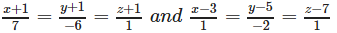Solution:

On comparing the given equations with :
In the cartesian form two lines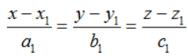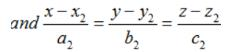we get ;

x1 = -1, y1 = -1,z1 = -1, ; a1 = 7, b1 = -6, c1 = 1 and

x2 = 3, y2 = 5, z2 = 7; a2 = 1, b2 = -2, c2 = 1

Now the shortest distance between the lines is given by :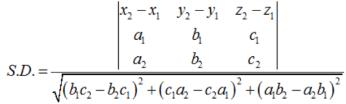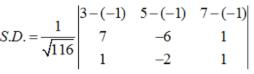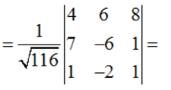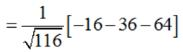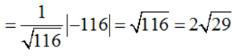QUESTION: 4

The angle θ between the planes A1x + B1y + C1z + D1 = 0 and A2 x + B2 y + C2 z + D2 = 0 is given by

Solution:

By definition , The angle θ between the planes A1x + B1y + C1z + D1 = 0 and A2 x + B2 y + C2 z + D2 = 0 is given by :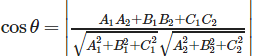QUESTION: 5

Find the equation of the plane through the line of intersection of the planes x + y + z = 1 and 2x + 3y + 4z = 5 which is perpendicular to the plane x – y + z = 0.

Solution:

The equation of the plane through the line of intersection of the planes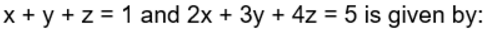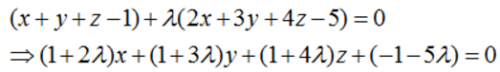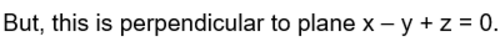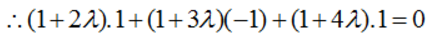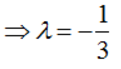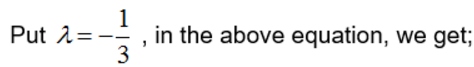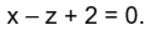QUESTION: 6

If l, m, n are the direction cosines of a line, then

Solution:

If l, m , n are the direction cosines of a line then , we know that,  l2+ m2+ n2 = 1.

QUESTION: 7

Shortest distance between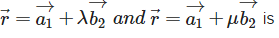Solution: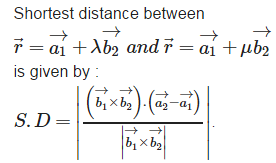QUESTION: 8

Find the shortest distance between the lines :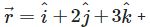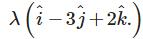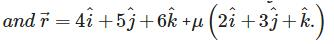Solution:

On comparing the given equations with: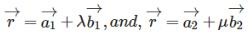, we get: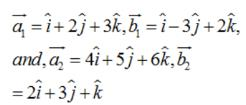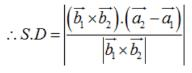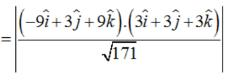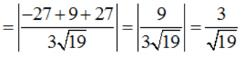QUESTION: 9

The distance of a point whose position vector is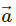from the plane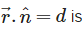Solution:

The distance of a point whose position vector isfrom the planegiven by :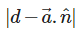QUESTION: 10

Find the angle between the planes whose vector equations are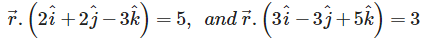Solution: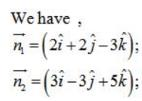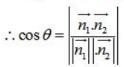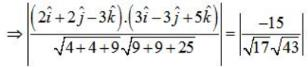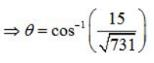QUESTION: 11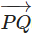is a vector joining two points P(x1, y1, z1) and Q(x2, y2, z2). If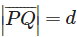Direction cosines ofare

Solution:is a vector joining two points P(x1, y1, z1) and Q(x2, y2, z2). IfDirection cosines ofare given by :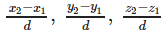QUESTION: 12

Shortest distance between the lines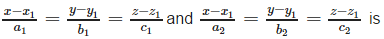Solution:

In Cartesian coordinate system Shortest distance between the lines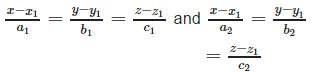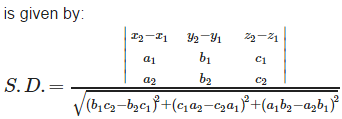QUESTION: 13

Find the shortest distance between the lines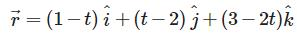and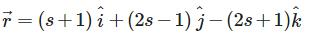Solution:

Find the shortest distance between the lines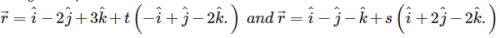On comparing them with :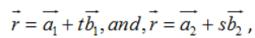we get :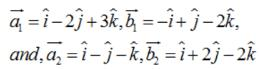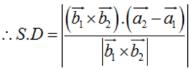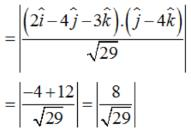QUESTION: 14

The distance d from a point P(x1, y1, z1) to the plane Ax + By + Cz + D = 0 is

Solution:

The distance d from a point P(x1, y1, z1) to the plane Ax + By + Cz + D = 0 is given by :

QUESTION: 15

Determine whether the given planes are parallel or perpendicular, and in case they are neither, find the angles between them.7x + 5y + 6z + 30 = 0 and 3x – y – 10z + 4 = 0

Solution: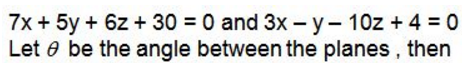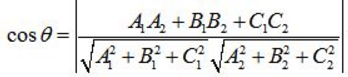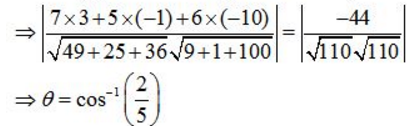QUESTION: 16

If l, m and n are the direction cosines of a line, Direction ratios of the line are the numbers which are

Solution:

If l, m and n are the direction cosines of a line, Direction ratios of the line are the numbers which are Proportional to the direction cosines of the line.

QUESTION: 17

Distance between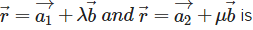Solution:

In vector form Distance between two parallel linesgiven by :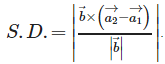QUESTION: 18

Find the angle between the following pairs of lines: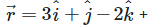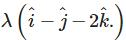and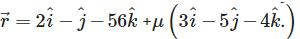Solution:

If θ is the acute angle between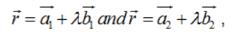then cosine of the angle between
these two lines is given by :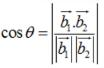Here,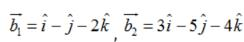Then,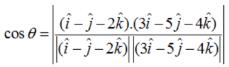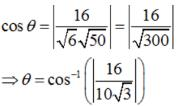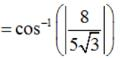QUESTION: 19

Determine the direction cosines of the normal to the plane and the distance from the origin. Plane z = 2

Solution:

We have z = 2 . , it can be written as : 0x+0y+1z = 2. Compare it with lx+my+nz = d , we get ; l = 0 , m = 0 , n = 1 and d = 2 . therefore , D.C.’s of normal to the plane are 0 , 0 , 1 and distance from the origin = 2.

QUESTION: 20

In the following case, determine whether the given planes are parallel orperpendicular, and in case they are neither, find the angles between them. 2x + y + 3z – 2 = 0 and x – 2y + 5 = 0

Solution:

We have ,
2x + y + 3z – 2 = 0 and x – 2y + 5 = 0. Let θ be the angle between the planes , then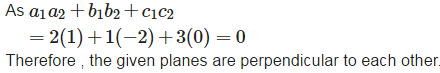QUESTION: 21

If l, m, n are the direction cosines and a, b, c are the direction ratios of a line then

Solution:

If l, m, n are the direction cosines and a, b, c are the direction ratios of a line then , the directions cosines of the line are given by :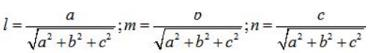QUESTION: 22

If a line makes angles 90, 135, 45 with the x, y and z – axes respectively, find its direction cosines.

Solution:

If a line makes angles 90, 135, 45 with the x, y and z – axes respectively, then the direction cosines of this line is given by :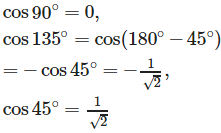QUESTION: 23

In the vector form, equation of a plane which is at a distance d from the origin, and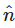is the unit vector normal to the plane through the origin is

Solution:

In the vector form, equation of a plane which is at a distance d from the origin, andis the unit vector normal to the plane through the origin is given by :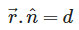QUESTION: 24

Determine the direction cosines of the normal to the plane and the distance from the origin. Plane x + y + z = 1

Solution:

Here , D.R’s of normal to the plane are 1, 1 , 1 ,its D.C ‘s are :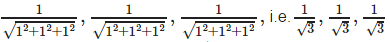On dividing x + y + z = 1 by √3 , we get :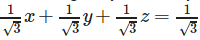It is of the form : lx+my+nz = d , therefore , d = 1/√3 .

QUESTION: 25

In the following case, determine whether the given planes are parallel or perpendicular, and in case they are neither, find the angles between them. 2x – 2y + 4z + 5 = 0 and 3x – 3y + 6z – 1 = 0

Solution: